# Chapter 23 Graphical Representation of Statistical Data RD Sharma Solutions Exercise 23.1 Class 9 Maths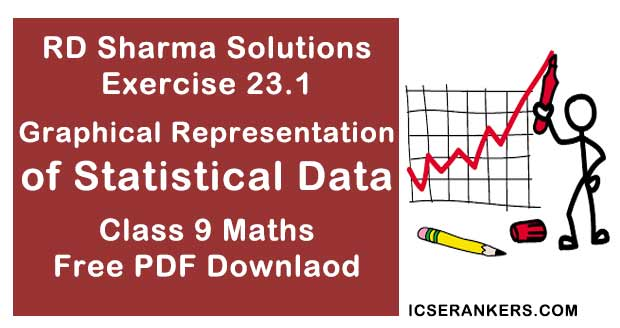Chapter Name RD Sharma Chapter 23 Tabular Representation of Statistical Data Exercise 23.1 Book Name RD Sharma Mathematics for Class 10 Other Exercises Exercise 23.2 Related Study NCERT Solutions for Class 10 Maths

### Exercise 23.1 Solutions

1. Read the bar graph shown in Fig. 23.8 and answer the following question :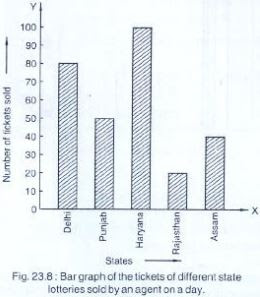(i) What is the information given by the bar graph?
(ii) How many tickets of Assam State Lottery were sold by the agent?
(iii) Of which state, were the maximum number of tickets sold?
(iv) State whether true or false.
The maximum number of tickets sold is three times the minimum number of tickets
sold.
(v) Of which state were the minimum number of tickets sold?
Solution
(i) The given bar graph represents the number of the tickets of different state lotteries sold
by an agent on a day

(ii) Number of tickets of Assam state lottery were sold by agent is 40

(iii) Haryana sold the maximum number of tickets

(iv) Minimum number of tickets sold = 20
Maximum number of tickets sold = 100
∴ 100 = 5 ×20
So, the given statement is false

(v) Rajasthan sold the minimum number of tickets.

2.Studey the bar graph representing the number of persons in various age groups in a town shown in Fig. below. Observe the bar graph and answer the following questions:
(i) What is the percentage of the youngest age-group persons over those in the oldest age
group?
(ii) What is the total population of the town?
(iii) What is the number of persons in the age group 60 - 65?
(iv) How many persons are more in the age-group 10 - 15 than in the age group 30 - 35?
(v) What is the age-group of exactly 1200 persons living in the town?
(vi) What is the total number of persons living in the town in the age-group 50 - 55?
(vii) What is the total number of persons living in the town in the age-groups 10 - 15 and 60 - 65?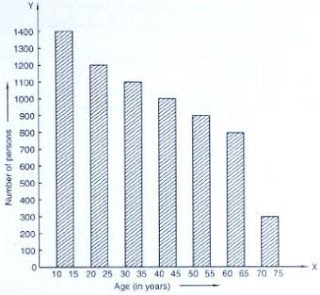(viii) Whether the population in general increases, decreases or remains constant with the
increase in the age-group.
Solution
(i) The percentage of the youngest age group persons over those in the oldest age group
= 1400/300 ×100  = 1400/3

(ii) Total population of the town
= 1400 + 1200 + 1100 + 1000 + 900 + 800 + 300 = 6700

(iii) The number of persons in the age group of 60 - 65 is 800.

(iv) The number of persons are more in the age group 10 - 15 than in the age group 30-35
= 1400 - 1100 = 300

(v) The age group in which exactly 1200 persons living in the town is 20 - 25

(vi) The total number of person living in the town is 20-25

(vii) The total number of persons living in the town in the age group 10 to 15 and 60-65
= 1400 + 800 = 2,200

(viii) The population decreases with the increase in the group.

3. Read the bar graph shown in Fig. 23.10 and answer the following questions
(i) What is the information given by the bar graph?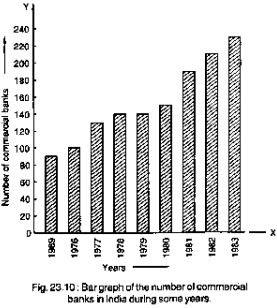(ii) What was the number of commercial banks in 1977?
(iii) What is the ratio of the number of commercial banks in 1969 to that in 1980?
(iv) State whether true or false:
The number of commercial banks in 1983 is less than double the number of
commercial banks in 1969.
Solution
(i) Given bar graph represents the number of commercial banks in India during some
years.

(ii) The number of commercial banks in 1977 was 130.

(iii) The ratio of the number of commercial banks in 1969 to that in 1980 = 90/150 = 3 : 5

(iv) The number of commercial banks in 1983 = 230
The number of commercial banks in 1980 = 150
Clearly, the number of commercial banks in 1983 is not less than double the number of commercial banks in 1969.
So, the given statement is false.

4. Given below (Fig. below) is the bar graph indicating the marks obtained out of 50 in
mathematics paper by 100 students. Read the bar graph and answer the following questions: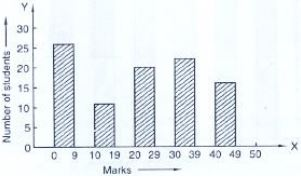(i) It is decided to distribute work books on mathematics to the students obtaining
less than 20 marks, giving one workbook to each of such students. If a work book costs Rs 5, what sum is required to buy the work books?
(ii) Every student belonging to the highest mark group is entitled to get a prize of Rs. 10.
How much amount of money is required for distributing the prize money?
(iii) Every student belonging to the lowest mark—group has to solve 5 problems per day.
How many problems, in all, will be solved by the students of this group per day?
(iv) State whether true or false.
a. 17% students have obtained marks ranging from 40 to 49.
b. 59 students have obtained marks ranging from 10 to 29.
(v) What is the number of students getting less than 20 marks?
(vi) What is the number of students getting more than 29 marks?
(vii) What is the number of students getting marks between 9 and 40?
(viii) What is the number of students belonging to the highest mark group?
(ix) What is the number of students obtaining more than 19 marks?
Solution
(i) Total number of students obtaining less than 20 marks = 27 + 12 = 39
The cost off one - work book = Rs 5.
∴ The cost of 30 work books = 39 × 5
= Rs. 195.

(ii) The number of students belonging to the highest mark group = 17
The cost of a prize = 10.
∴ The cost of 17 prizes = 10 × 7
= Rs. 170

(iii) The number of students belonging to the lowest mark group = 27
The number of problems solved by 1 student = 5
∴ The total number of problems solved by 27 students = 5 × 27 = 135.

(iv) (a) Total number of students = 100
The number of students in range = 40 - 49 = 17.
% of students obtaining marks ranging
40 - 49 = 17/100 × 100 = 17%
So, the given statement is true.
(b) The given statement is false (False).

(v) No. of students getting more than 20 marks = 39

(vi) Total no. of students getting more than 29 marks = 41

(vii) The number of students getting marks between 9 and 40 = 12 + 20 + 24  = 56

(viii) The number of students belonging to the highest mark group = 17.

(ix) The number of students obtaining more than 19 marks = 100 - 27 - 12 = 61

5. Read the following bar graph (Fig. 23.12) and 23.12) and answer the following questions:
(i) What is the information given by the bar graph?
(ii) State each of the following whether true or false.
a. The number of government companies in 1957 is that of 1982 is 1 :9.
b. The number of government companies have decreased over the year 1957 to 1983.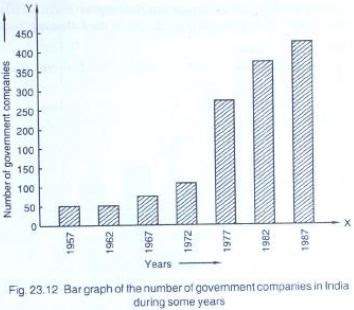Solution
(i) The given Bar graph represents the number of government companies in India during
some years.

(ii) (a) no. of government companies in 1957=50
Number of government companies in 1982=375
∴The number of government companies in 1957 is that of 1982 = 50/375
= 2/15 ≠ 1/9
So, the given statement is false
(b) The height of the bars increases over the years hence, the statement is false.

6. Read the following bar graph and answer the following questions :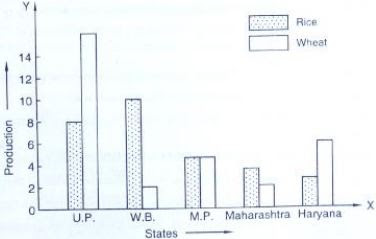(i) What information is given by the bar graph?
(ii) Which state is the largest producer of rice?
(iii) Which state is the largest producer of wheat?
(iv) Which state has total production of rice and wheat as its maximum?
(v) Which state has the total production of wheat and rice minimum?
Solution
(i) The given information is about rice and wheat production in various states of India.

(ii) W.B is the largest producer of rice

(iii) U.P is the largest producer of wheat

(iv) The total production of rice and wheat is maximum in U.P

(v) The total production of rice and wheat is minimum in Maharashtra

7. The following bar graph (Fig. 23. 1 4) represents the heights (in cm) of 50 students of Class XI of a particular school. Study the graph and answer the following questions: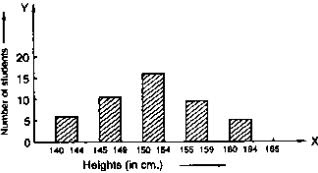(i) What percentage of the total number of students have their heights more than 149cm?
(ii) How many students in the class are in the range of maximum height of the class?
(iii) The school wants to provide a particular type of tonic to each student below the height
of 150 cm to improve his height. If the cost of the tonic for each student comes out
to be Rs. 55, how much amount of money is required?
(iv) How many students are in the range of shortest height of the class?
(v) State whether true or false:
a. There are 9 students in the class whose heights are in the range of 155 - 159 cm.
b. Maximum height (in cm) of a student in the class is 17.
c. There are 29 students in the class whose heights are in the range of 145- 154 cm.
d. Minimum height (in cm) of a student is the class is in the range of 140 – 144 cms.
e. The number of students in the class having their heights less than 150 cm is 12.
f. There are 14 students each of whom has height more than 154. cm.
Solution
(i) Total number of students have their heights more than 149 cm = 16 + 10 + 5 = 31 .
The percentage of the total number of students has their heights more than 149 cm
= 31/50 × 100 = 31 × 2 = 62 %

(ii) The number of students in the range of maximum height of the class is 5.

(iii) Total number of students below height of 150cm  = 7 + 12 = 19
The cost of the tonic for each student = Rs. 55
The cost of the tonic for 19 student = 19 × 55  = Rs 1045

(iv) The number of students are in the range of shortest height of the class = 7.

(v) (a) True
(b) False
(c) Total number of students in the range of 145 - 154
= 12 + 17 = 29
So, the given statement is true.
(d) True
(e) The number of students whose height more than 154 cm = 9 + 5 = 14.
(f) So, the given statement is true.

(i) What information is given by the bar graph?
(ii) What was the production of a student in the year 1980 - 81?
(iii) What is the minimum and maximum productions of cement and corresponding years?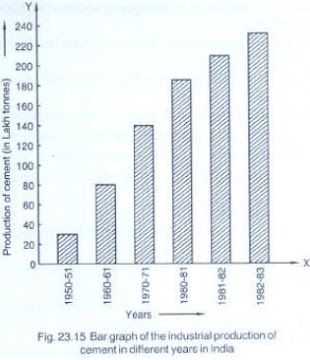Solution
(i) It gives information regarding industrial production of cement in different years in
India.

(ii) The production of cement in the year 1980 – 1981 = 186 lakh tones.

(iii) The minimum production is 30 lakh tones in 1950 – 1951 and maximum production 232 lakh tones in 1982–1983.

9. The bar graph shown in Fig 23.16 represents the circulation of newspapers in 10 languages.
Study the bar graph and answer the following questions: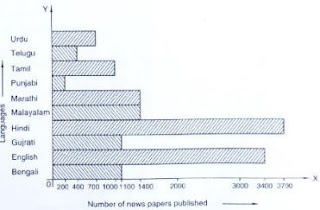(i) What is the total number of newspapers published in Hindi, English, Urdu, Punjabi and Bengali?
(ii) What percent is the number of news papers published in Hindi of the total number of
newspapers?
(iii) Find the excess of the number of newspapers published in English over those published in Urdu.
(iv) Name two pairs of languages which publish the same number of newspapers.
(v) State the language in which the smallest number of newspapers are published.
(vi) State the language in which the largest number of newspapers are published.
(vii) State the language in which the number of newspapers published is between 2500 and 3500.
(viii) State whether true or false:
a. The number of newspapers published in Malayalam and Marathi together is less than those published in English.
b. The number of newspapers published in Telugu is more than those published in Tamil.
Solution
(i) Total number of newspapers published in Hindi, English, Urdu, Punjabi and Bengali
= 3700 + 3400 + 700 + 200 + 1100 = 9100.

(ii) The number of newspapers published in Hindi = 3700
The total number of newspaper is published
= 700 + 400 + 1000 + 200 + 1400 + 1400 + 700 + 1100 + 3400 + 1100 = 14, 400
The percentage of Hindi news papers 3700/14400 × 100 = 25.69 = 25.71

(iii) The total number of newspaper are published = 700 + 400 + 1000 + 200 + 1500 + 1400 + 3700 + 1100 + 3400 + 1100 = 14400
The percentage of Hindi news paper 3700/14400 × 100 = 25.69 = 25.71 %

(iv) Bengali, Gujrati, and Marathi, Malayalam are the two pairs of languages which publish the same number of newspaper.
(v) Punjabi is the Languages in which the smallest number of newspaper were published.

(vi) Hindi is the language in which the largest numbers of newspaper were published.

(vii) English is the language in which the number of newspaper were published in between 2500 and 3500

(viii) (a) Total number of newspaper were published in Malayalam and Marathi
= 1400 + 1400 = 2800.
Number of newspaper were published in English = 3400
∴The number of newspapers published in Malayalam and Marathi together is less than those published in English so the given statement is true.
(b) Number of news. Papers published in Telugu = 400
Number of newspaper published in Tamil = 1000
∴The number of newspapers published in Telugu is more less than those published in
Tamil.
So, the given statement is false.

10. Read the bar graph given in Fig. 23.17 and  answer the following questions:
(i) What information is given by the bar graph?
(ii) What was the crop-production of rice in 1970 - 71?
(iii) What is the difference between the maximum and minimum production of rice?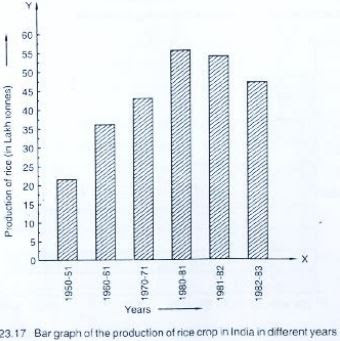Solution
(i) It gives information regarding the production of rice crop in India in different years
(ii) The crop production of rice in 1970 - 71 = 42.5 lakh tonnes.
(iii) The difference between the maximum and minimum production of rice = 55 – 22 = 33 lakh tonnes

11. Read the bar graph given in Fig. below and answer the following question ?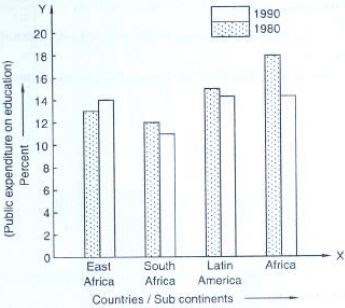(i) What information does it give?
(ii) In which part the expenditure on education is maximum in 1980?
(iii) In which part the expenditure has gone up from 1980 to 1990?
(iv) In which part the gap between 1980 and 1990 is maximum?
Solution
(i) It gives the information about the public expenditure one education by various state
subcontinents
(ii) In Africa the expenditure education is maximum in 1980
(iii) In east Africa. The expenditure has gone by from 1980 to 1990
(iv) In Africa the gap between 1980 and 1990 is maximum.

12. Read the bar graph given in Fig. 23.19 and answer the following questions:
(i) What information is given by the bar graph?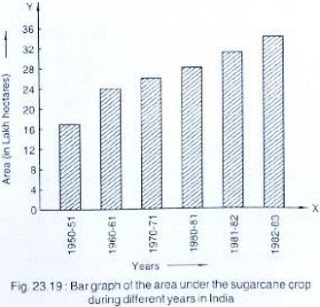(ii) In which years the areas under the sugarcane crop were the maximum and the minimum?
(iii) State whether true or false:
The area under the sugarcane crop in the year 1982 - 83 is three times that of the year 1950 - 51
Solution
(i) It gives the information about the areas under sugarcane crop during different years in India

(ii) The areas under the sugarcane crop were the maximum and the minimum in 1982 - 1983 and 1950 - 51 respectively

(iii) The area under sugarcane crop in the year 1982 - 1983 = 34 lakhs hectors.
The area under sugarcane crop in the year 1950 - 51 = 17 lakh hectors
Clearly, the area under the sugarcane crop in the year 1982 - 83 is not three tomes that of the year 1950 - 51
So, the given statement is false

13. Read the bar graph given in Fig. 23.20 and answer the following questions: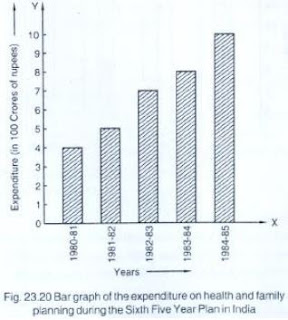(i) What information is given by the bar graph?
(ii) What was the expenditure on health and family planning in the year 1982-83?
(iii) In which year is the increase in expenditure maximum over the expenditure in previous year? What is the maximum increase?
Solution
(i) It gives the information about the expenditure on health and family planning during sixth five plan in India

(ii) The expenditure on health and many family planning in the year 1982 - 83 = Rs 700 cores

(iii) 1984 - 85 is the year in which the increase in expenditure maximum over the expenditure in previous year.
The maximum increase = 1000 - 780 = 220 crores.

14. Read the bar graph given in Fig. 23.21 and answer the following questions :
(i) What is the information given by the bar graph?
(ii) What is the number of families having 6 members?
(iii) How many members per family are there in the maximum number of families? Also tell the number of such families.
(iv) What are the number of members per family for which the number of families are equal? Also, tell the number of such families?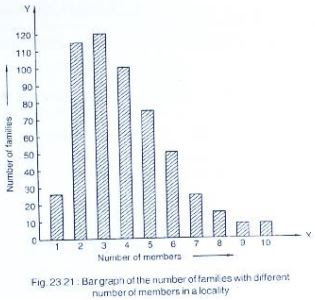Solution
(i) It gives the information about the number of families with different number of members in a locality.

(ii) The number of families having 6 members = 50

(iii) 3 members per family are there in the maximum number of families
The number of families which have 3 members = 120.

(iv) 9 and  10 are the number of members per family for which the number of families are equal
The number of such families is 5.

15. Read the bar graph given in Fig. 23.22 and answer the following questions: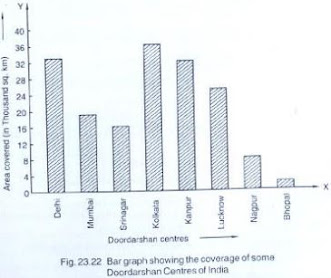(i) What information is given by the bar graph ?
(ii) Which Doordarshan centre covers maximum area ? Also tell the covered area.
(iii) What is the difference between the areas covered by the centres at delhi and Bombay?
(iv) Which Doordarshan centres are in U.P State? What are the areas covered by them?
Solution
(i) It gives the information above the overage of some Door Darshan centers of India

(ii) Kolkata Door Darshan center convers maximum area.
The area covered by Kolkata door darshan
Centre = 36000 sq - km

(iii) The difference between the areas covered by the centers of Delhi and Bombay
= 33000 - 19000
= 14000 sq - km

(iv) Kanpur and Lucknow doordarshan center are in U.P state
The area covered by Kanpur doordarshan center = 32000 sq km
The area covered by Lucknow doordarshan center = 25000 sq km